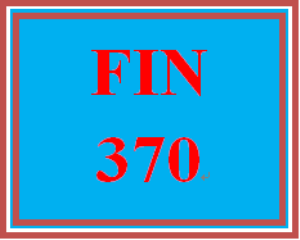# FIN 370T Wk 3 – Practice: Ch. 7 and 8 Knowledge Check

PLDZ-15373 Free In Stock
\$ 0.00 USD
Description

https://hwsell.com/category/fin-370/

~~~~~~~~~~~~~~~~~~~~~~~~~~~~~~

https://www.hwsell.com/

## FIN 370T Wk 3 – Practice: Ch. 7 and 8 Knowledge Check

Bond prices are quoted in terms of which of the following?

Multiple Choice

•      Original issue discount
•      Percent of par value
•      Coupon rate in dollars
•      Market rate in dollars

Consider the following bond quote: a municipal bond quoted at 101.25. If the municipal bond has a par value of \$5,000, what is the price of the bond in dollars?

Multiple Choice

•      \$5,089.06
•      \$5,050.19
•      \$5,062.50
•      \$5,109.75

A 3.75 percent TIPS has an original reference CPI of 183.9. If the current CPI is 214.7, what is the current interest payment? (Assume semi-annual interest payments and a par value of \$1,000.)

Multiple Choice

•      \$43.78
•      \$37.50
•      \$21.89
•      \$18.75

Determine the interest payment for the following three bonds: 2.5 percent coupon corporate bond (paid semi-annually), 3.15 percent coupon Treasury note, and a corporate zero coupon bond maturing in 10 years. (Assume a \$1,000 par value.)

Multiple Choice

•      \$2.50, \$3.15, \$0, respectively
•      \$12.50, \$15.75, \$0, respectively
•      \$12.50, \$15.75, \$100, respectively
•      \$25.00, \$31.50, \$0, respectively

A 3.25 percent TIPS has an original reference CPI of 194.1. If the current CPI is 210.3, what is the current interest payment? (Assume semi-annual interest payments and a par value of \$1,000.)

Multiple Choice

•      \$15.00
•      \$16.25
•      \$17.61
•      \$31.54

Bonds are issued by which of the following?

Multiple Choice

•      Corporations
•      Federal government or its agencies
•      State and local governments
•      All of the options

Regarding a bond's characteristics, which of the following is the principal loan amount that the borrower must repay?

Multiple Choice

•      Maturity date
•      Par or face value
•      Time to maturity value

Which of the following terms means the chance that future interest payments will have to be reinvested at a lower interest rate?

Multiple Choice

•      Credit quality risk
•      Interest rate risk
•      Liquidity rate risk
•      Reinvestment rate risk

What's the current yield of an 8.15 percent coupon corporate bond quoted at a price of 94.30?

Multiple Choice

•      4.30 percent
•      8.01 percent
•      8.15 percent
•      8.64 percent

Rank the following bonds in order from lowest credit risk to highest risk all with the same time to maturity, by their yield to maturity: JM Corporate bond with yield of 12.25 percent, IB Corporate bond with yield of 4.49 percent, TC Corporate bond with yield of 8.76 percent, and B&O Corporate bond with a yield of 5.99 percent.

Multiple Choice

•      JM bond, TC bond, B&O bond, IB bond
•      IB bond, B&O bond, TC bond, JM bond
•      TC bond, B&O bond, IB bond, JM bond
•      JM bond, IB bond, B&O bond, TC bond
Tags
Recent Reviews Write a Review
0 0 0 0 reviews